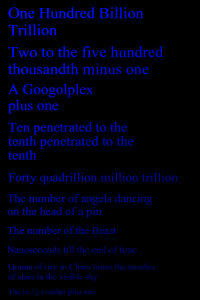# The Berry NumberImagine the smallest integer which is too large to be described in, say, less than two hundred words. When we refer to describing a number in words, we mean a proper English construct which logically must refer to a specific finite integer. Examples would include common mathematical language, such as "Two raised to the five hundredth power, plus one." Also acceptable would be more abstract descriptions which still describe unique integers, such as "The number of molecules of water in the Indian Ocean."

Obviously this number could be quite large, since with the flexibility of our naming convention for numbers we can describe huge numbers in a small space. (E.g., ten penetrated to the tenth describes a truly unimaginably large number) There are other numbers which are smaller but require more words. For example, one thousand, four-hundred twenty-seven requires six words. The first integer which cannot be described in less than two hundred words is called the Berry Number.

Can you find, or give a logical demonstration for the non-existence of, this number?